Magnetism Exam1 and Problem Solutions

Magnetism Exam1 and  Problem Solutions

1. Find the forces exerted by S poles of magnets given below.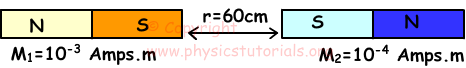F=k.M1.M2/r2=(10-7.10-4.10-3)/(0,6)2

F=10-14/(36.10-2)

F=10-12/36

2. Find resultant magnetic field at point O, produced by I1, I2 and I3.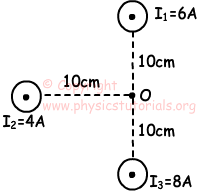Magnitudes of magnetic fields;

B1=2k.6/0,1=12.10-7/10-1=12.10-6 N/Amps.m

B2=2k.4/0,1=8.10-7/10-1=8.10-6 N/Amps.m

B3=2k.8/0,1=16.10-7/10-1=16.10-6 N/Amps.m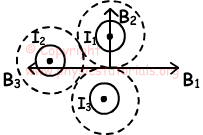Bresultant=B1+B2+B3

Bresultant=√(12.10-6-16.10-6)2+(8.10-6)2

Bresultant=4√5.10-6 N/Amps.m

3. A, B and C wires are given below. Find the magnetic field of A, B and C at points X and Y.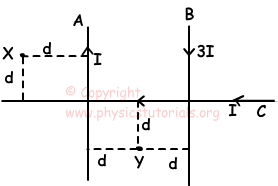Directions of magnetic fields at point X are found using right hand rule.

BA: outward

BB:inward

BC:inward

BX=BB+BC-BA

BX=2k.3I/3d+2k.I/d-2k.I/d=2k.I/d

Directions of magnetic fields at point Y are;

BA: inward

BB:inward

BC:outward

BY=BA+BB-BC

BY=2k.I/d+2k.3I/d-2k.I/d=2k.3I/d

Ratio of magnetic fields;

BX/BY=2k.I/d/2k.3I/d=1/3

4. Solenoid having number of loops N and surface area A is shown in picture given below. If we change the position of solenoid as shown in the picture below, find the equation used for finding induced emf of solenoid.Induced emf=ε=-(∆Φ)/(∆t).N

Change in Flux;

∆Φ=Φ21

Φ1=0, since cross section area of solenoid and magnetic field lines are parallel to each other.

Φ2=B.A

∆Φ=B.A-0=B.A

ε=-B.A.N/t

5. Draw the directions of magnetic field lines at point A, B, C and D in the picture given below.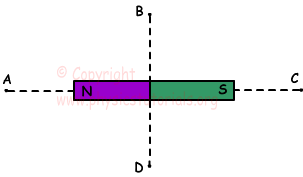Directions of magnetic field lines are drawn from N pole to S pole as shown in the picture given below.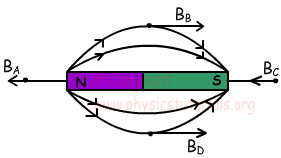Author: